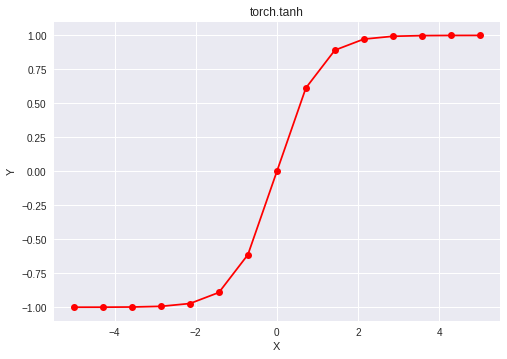GeeksforGeeks App
Open AppBrowser
Continue

## Related Articles

PyTorch is an open-source machine learning library developed by Facebook. It is used for deep neural network and natural language processing purposes.
One of the many activation functions is the hyperbolic tangent function (also known as tanh) which is defined as.
The hyperbolic tangent function outputs in the range (-1, 1), thus mapping strongly negative inputs to negative values. Unlike the sigmoid function, only near-zero values are mapped to near-zero outputs, and this solves the “vanishing gradients” problem to some extent. The hyperbolic tangent function is differentiable at every point and its derivative comes out to be. Since the expression involves the tanh function, its value can be reused to make the backward propagation faster.
Despite the lower chances of the network getting “stuck” when compared with the sigmoid function, the hyperbolic tangent function still suffers from “vanishing gradients”. Rectified Linear Unit (ReLU) can be used to overcome this problem.
The function torch.tanh() provides support for the hyperbolic tangent function in PyTorch. It expects the input in radian form and the output is in the range [-∞, ∞]. The input type is tensor and if the input contains more than one element, element-wise hyperbolic tangent is computed.

Syntax: torch.tanh(x, out=None)
Parameters
x: Input tensor
name (optional): Output tensor
Return type: A tensor with the same type as that of x.

Code #1:

## Python3

 `# Importing the PyTorch library``import` `torch` `# A constant tensor of size 6``a ``=` `torch.FloatTensor([``1.0``, ``-``0.5``, ``3.4``, ``-``2.1``, ``0.0``, ``-``6.5``])``print``(a)` `# Applying the tanh function and``# storing the result in 'b'``b ``=` `torch.tanh(a)``print``(b)`

Output:

``` 1.0000
-0.5000
3.4000
-2.1000
0.0000
-6.5000
[torch.FloatTensor of size 6]

0.7616
-0.4621
0.9978
-0.9705
0.0000
-1.0000
[torch.FloatTensor of size 6]```

Code #2: Visualization

## Python3

 `# Importing the PyTorch library``import` `torch` `# Importing the NumPy library``import` `numpy as np` `# Importing the matplotlib.pyplot function``import` `matplotlib.pyplot as plt` `# A vector of size 15 with values from -5 to 5``a ``=` `np.linspace(``-``5``, ``5``, ``15``)` `# Applying the hyperbolic tangent function and``# storing the result in 'b'``b ``=` `torch.tanh(torch.FloatTensor(a))` `print``(b)` `# Plotting``plt.plot(a, b.numpy(), color ``=` `'red'``, marker ``=` `"o")``plt.title("torch.tanh")``plt.xlabel("X")``plt.ylabel("Y")` `plt.show()`

Output:

```-0.9999
-0.9996
-0.9984
-0.9934
-0.9728
-0.8914
-0.6134
0.0000
0.6134
0.8914
0.9728
0.9934
0.9984
0.9996
0.9999
[torch.FloatTensor of size 15]```My Personal Notes arrow_drop_up